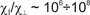Modern fusion devices (both stellarators and tokamaks) can have very general, 3-dimensional magnetic topologies in the plasma edge. It can include field lines contacting material surfaces, regions with intact magnetic surfaces and islands; special complexity is introduced by stochastic domains. Conventional methods of computational fluid dynamics are not applicable in this case directly: the problem is numerically stiff because of very high degree of anisotropy. One of the possible approaches to the modeling of heat and particles transport is Monte-Carlo. The "fluid" version of MC is based upon the fact that fluid transport equations are of the parabolic type like the ordinary diffusion equation. Therefore, one can use the analogy between the transport phenomena in plasmas and random walk of test particles which satisfies Fokker-Planck equation. The "kinetic" version is even more straightforward: the test particles here relate to physical particles, one follows them in real space and takes collisions into account, e.g., by means of random walk procedure. The advantages of the method are obvious: one can model transport phenomena in the magnetic field of (almost) any degree of complexity; in addition to this, MC approach can be naturally parallelized. The disadvantage is that in addition to usual numerical errors there is a special group of errors induced by noise (insufficient statistics).

Go to Editor View